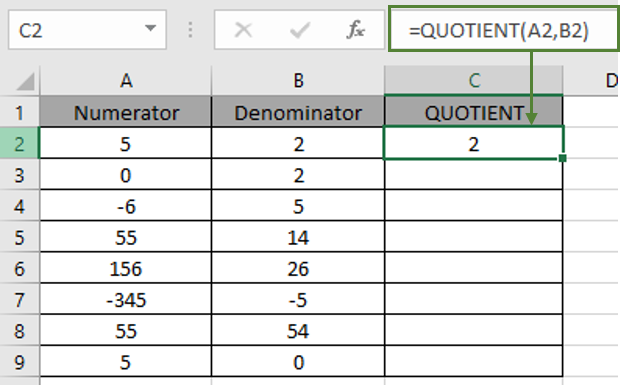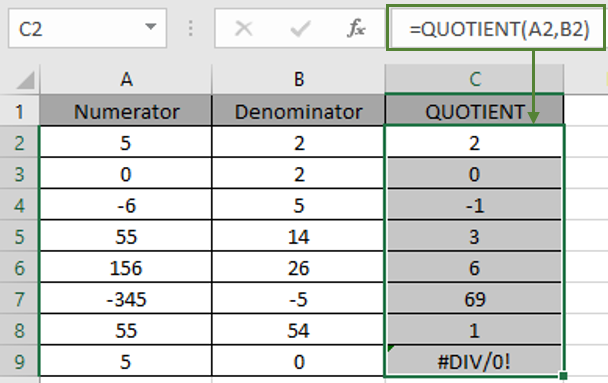# How to use the QUOTIENT function in Excel

The QUOTIENT function is a mathematical division function which returns the integer division of the numbers given in as input.

Dividend = Divisor * Quotient + Remainder

11 = 2 * 5 + 1

The QUOTIENT function takes two argument Dividend ( numerator ) and Divisor ( denominator ) adn returns the Integer Quotient without the remainder.

Syntax for the QUOTIENT function:

= QUOTIENT ( numerator, denominator )

numerator: number, dividend which is being divided
denominator: number, divisor which divides the dividend or the numerator

All of this might be confusing so let’s learn about the QUOTIENT function using in an example.

Here we have a list of values as Numerator and Denominator and using the Quotient function formula will get use the QUOTIENT for the numbers.

= QUOTIENT ( A2 , B2 )

Here A2 & B2 are given as cell reference as argument to the function.As you can see when 5 is divided by 2, Quotient comes out to be 2 and the remainder is 1.

5 = 2 * 2 + 1

Now copy the formula to other cells using the Ctrl + D shortcut or using the drop-down cell option in excel.The above snapshot clears some doubts about the QUOTIENT function. And you might be wondering about the #DIV/0 error in the C9 cell. When any number is divided by 0 i.e. number / 0, the function returns #DIV/0 error. Below are some of the observational results about the function.

Notes:

• The function returns the #DIV/0 error if the denominator argument of the function is 0 or blank cell (considered as value 0).
• The function returns the #VALUE! Error if the numerator argument is non - numeric.
• The function returns the #VALUE! Error if the denominator argument is non - numeric.
• The numerator can have the value 0 and returns Quotient as 0 for the same.
• The arguments numerator & denominator both can have any value either rational or irrational. ( Denominator cannot be 0 )

Hope this article about How to use the QUOTIENT function in Excel is explanatory. Find more articles on mathematical functions here. Please share your query below in the comment box. We will assist you.

Related Articles

Calculate a ratio of two numbers in Excel

How to use PRODUCT Function in Excel

Insert Division symbol in Excel

How to use the GCD Function in Excel

How to use the LCM Function in Excel

Popular Articles

Edit a dropdown list

Absolute reference in Excel

If with conditional formatting

If with wildcards

Vlookup by date

Join first and last name in excel

Count cells which match either A or B

Convert Inches To Feet and Inches in Excel 2016

50 Excel Shortcut to Increase Your Productivity

Terms and Conditions of use

The applications/code on this site are distributed as is and without warranties or liability. In no event shall the owner of the copyrights, or the authors of the applications/code be liable for any loss of profit, any problems or any damage resulting from the use or evaluation of the applications/code.